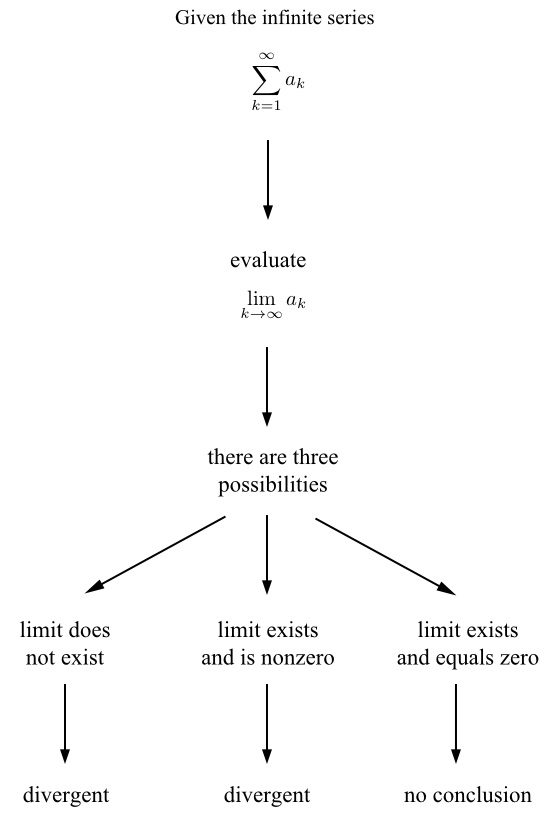# A Divergence Test Flowchart

Previous: The Divergence Test

Next: Simple Divergence Test Example

The steps involved in applying the divergence test to an infinite series are given in the flowchart below.This diagram shows the steps involved in conducting the divergence test. Observe that in the case that the limit equals zero, the test does not tell us if the series is convergent or divergent (other convergence tests can be employed in this case). This is an area that is a common source of confusion among calculus students.

Previous: The Divergence Test

Next: Simple Divergence Test Example